2020/11/30 13:33

### 1.项目介绍

下载安装命令

## CPU版本安装命令

## GPU版本安装命令
pip install -f https://paddlepaddle.org.cn/pip/oschina/gpu paddlepaddle-gpu

AI Studio项目地址：
https://aistudio.baidu.com/aistudio/projectdetail/1109418

## 2.前言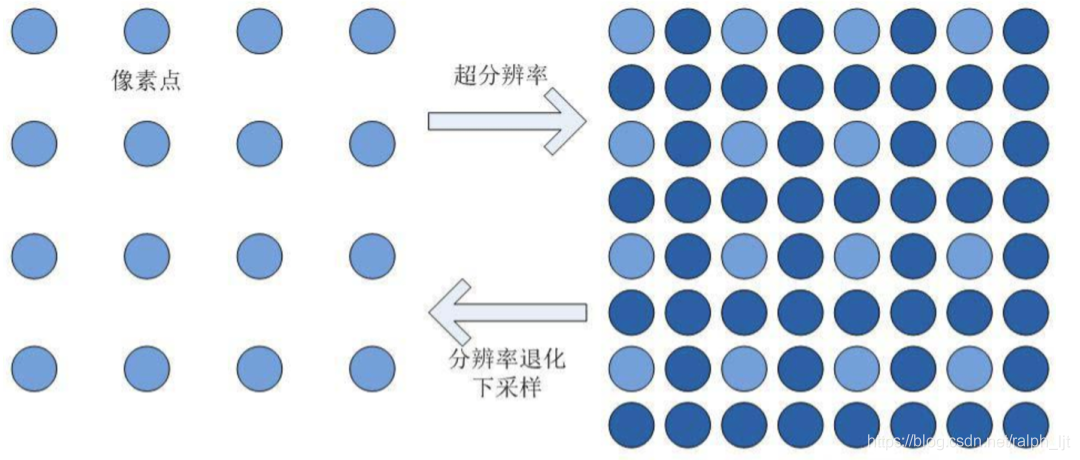SRCNN：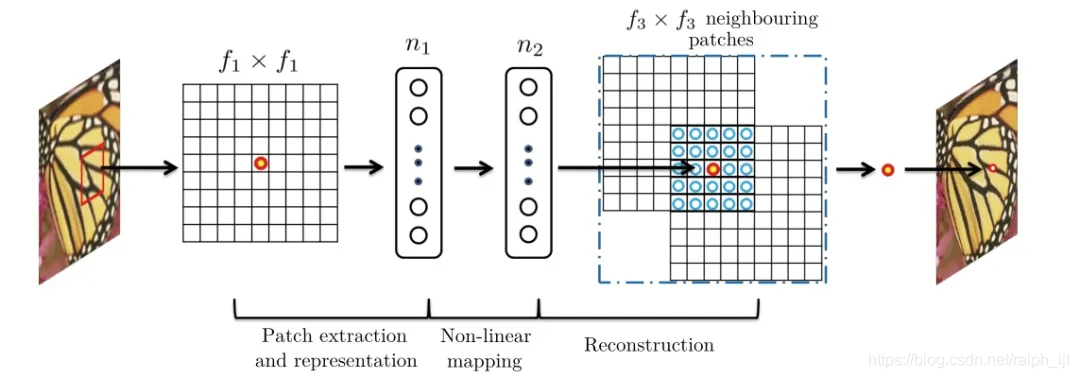SRGAN：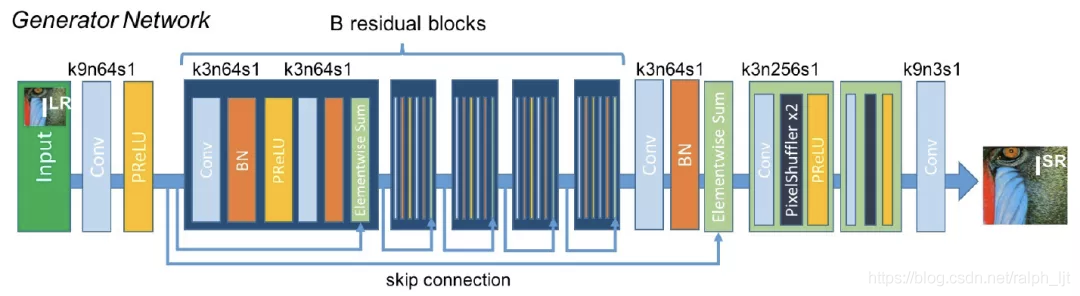DRN：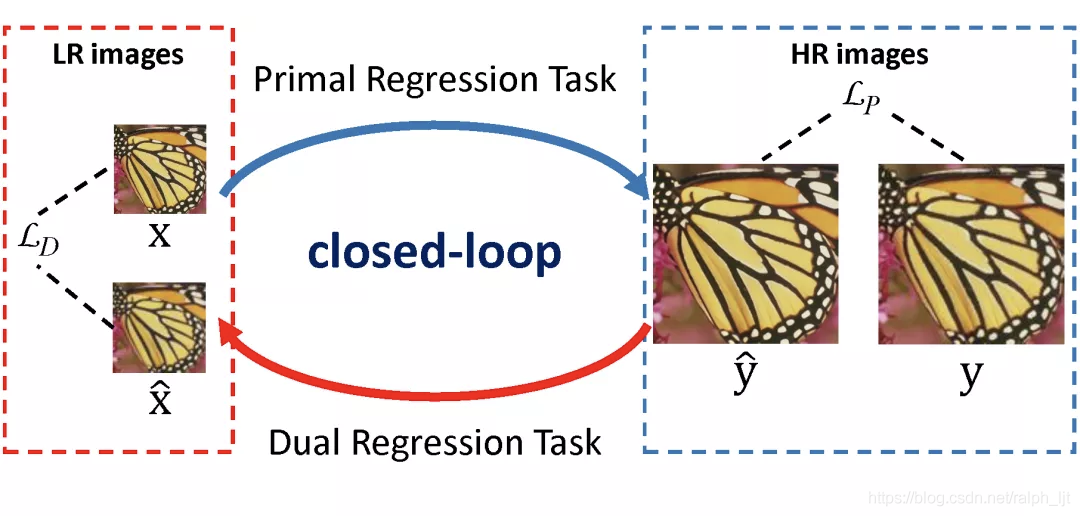USRNet: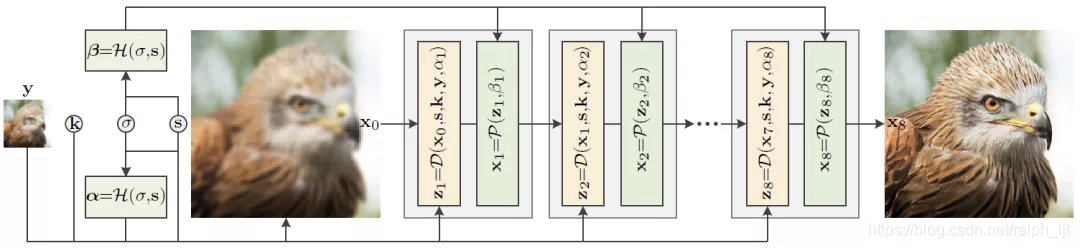### 3.项目背景

#### 3.2 网络结构：

https://aistudio.baidu.com/aistudio/projectdetail/1109418

#### 3.3.1 数据预处理

class BSD_data(Dataset):

def __init__(self,
mode='train',
image_path="data/data55873/images/"
):
super(BSD_data, self).__init__()

self.mode = mode.lower()
if self.mode == 'train':
self.image_path = os.path.join(image_path,'train')
elif self.mode == 'val':
self.image_path = os.path.join(image_path,'val')
else:
raise ValueError('mode must be "train" or "val"')

# 原始图像的缩放大小
self.crop_size = 300
# 缩放倍率
self.upscale_factor = 3
# 缩小后送入神经网络的大小
self.input_size = self.crop_size // self.upscale_factor
# numpy随机数种子
self.seed=1337
# 图片集合
self.temp_images = []
# 加载数据
self._parse_dataset()

def transforms(self, img):
"""
图像预处理工具，用于将升维(100, 100) => (100, 100,1)，
并进行维度转换 H W C => C H W
"""
if len(img.shape) == 2:
img = np.expand_dims(img, axis=2)
return img.transpose((2, 0, 1))

def __getitem__(self, idx):
"""
返回 缩小3倍后的图片 和 原始图片
"""
# 加载原始图像
# 将原始图像缩放到（3, 300, 300）
img = img.resize(
[self.crop_size,self.crop_size],
Image.BICUBIC
)

#转换为YCbCr图像
ycbcr = img.convert("YCbCr")

# 因为人眼对亮度敏感，所以只取Y通道
y, cb, cr = ycbcr.split()
y = np.asarray(y,dtype='float32')
y = y / 255.0

# 缩放后的图像和前面采取一样的操作
img_ = img.resize(
[self.input_size,self.input_size],
Image.BICUBIC
)
ycbcr_ = img_.convert("YCbCr")
y_, cb_, cr_ = ycbcr_.split()
y_ = np.asarray(y_,dtype='float32')
y_ = y_ / 255.0

# 升维并将HWC转换为CHW
img_s = self.transforms(y)
img_l = self.transforms(y_)

# img_s 为缩小3倍后的图片（1, 100, 100）
# img_l 是原始图片（1, 300, 300）
return img_s , img_l

def __len__(self):
"""
实现__len__方法，返回数据集总数目
"""
return len(self.temp_images)

def _sort_images(self, img_dir):
"""
对文件夹内的图像进行按照文件名排序
"""
files = []

for item in os.listdir(img_dir):
if item.split('.')[-1].lower() in ["jpg",'jpeg','png']:
files.append(os.path.join(img_dir, item))
return sorted(files)

def _parse_dataset(self):
"""
处理数据集
"""
self.temp_images = self._sort_images(self.image_path)
random.Random(self.seed).shuffle(self.temp_images)

"""
从磁盘读取图片
"""
with open(path, 'rb') as f:
img = img.convert('RGB')
return img



#### 3.3.2 定义网络结构:

from paddle.nn import Layer, Conv2D
class Sub_Pixel_CNN(Layer):

def __init__(self, upscale_factor=3, channels=1):
super(Sub_Pixel_CNN, self).__init__()
self.conv1 = Conv2D(channels,64,5,stride=1, padding=2)

self.conv2 = Conv2D(64,32,3,stride=1, padding=1)
self.conv3 = Conv2D(32,channels * (upscale_factor ** 2),3,stride=1, padding=1)

def forward(self, x):
x = self.conv1(x)
x = self.conv2(x)
x = self.conv3(x)
return x



#### 3.3.3 模型封装

model = paddle.Model(Sub_Pixel_CNN())



#### 3.3.4 模型可视化

model.summary((1, 1, 100, 100))

#### 3.3.5 模型训练准备

model.prepare(
learning_rate=0.001,
parameters=model.parameters()
),
)

#### 3.3.6 模型训练:

# 启动模型训练，指定训练数据集、训练轮数、批次大小、日志格式
model.fit(train_dataset,
epochs=1,
batch_size=16,
verbose=1)

#### 3.3.7 结果可视化

psmr_low: 30.381882136539197 psmr_pre: 29.4920122281961

### 4 .思考与总结

下载安装命令

## CPU版本安装命令

## GPU版本安装命令


juntao.lu@connect.qut.edu.au

GitHub:
Gitee:

### 飞桨官网地址:### 作者的其它热门文章

0
1 收藏

0 评论
1 收藏
0